HOMEWORK SOLUTION

HW #3

GEOTECHNICAL ENGINEERING I

(Question no. 3.22-d,f, 3.24-b,e, 3.25-b,e……Al-Khafaji textbook)3.22d   % passing #4                = 80                 % retained #4               = 100-80 = 20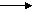% passing #200            = 20                 % retained (#4-#200)   = 80-20   = 60

LL                                = 40

PL                                = 32

PI                                 = 40-42=8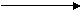< 50% passing #200                             coarse grained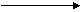< 50% retained #4                                sand> 12% passing #200                             use plasticity chart by plotting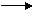LL = 40 and PI= 8                   ML

Then the soil is SM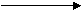≥ 15% gravel                                        Silty Sand with Gravel  ….  Ans.3.22d   % passing #4                = 87                 % retained #4               = 100-87 = 13% passing #200            = 40                 % retained (#4-#200)   = 87-40   = 47

LL                                = 62

PL                                = 25

PI                                 = 62-25=37< 50% passing #200                             coarse grained< 50% retained #4                                sand> 12% passing #200                             use plasticity chart by plottingLL = 62 and PI= 37                 CH

Then the soil is SC≥ 15% gravel                                        Clayey Sand  ….  Ans.3.22d   % passing #4                = 74                 % retained #4               = 100-74 = 26% passing #200            = 12                 % retained (#4-#200)   = 74-12   = 62

LL                                = 19

PL                                = 12

PI                                 = 19-12=7D60 = 2.34

D30 = 0.48                  interpolation

D10 = 0.034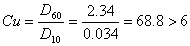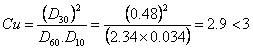< 50% passing #200                             coarse grained< 50% retained #4                                sand5-12% passing #200                            has double symbol

Since Cu > 6 and Cc 1≤Cc≤3 and using plasticity chart by plotting LL &PICL – ML

Then the soil is SW – SC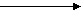≥15% gravel                             Well graded sand with clay and gravel

( or silty clay and gravel) …………….ans.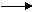3.24e   % passing #4      = 100             % retained #4               = 100-100 = 26% passing #200 = 4                  % retained (#4-#200)   = 100-4   = 96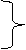D60 = 1.87

D30 = 0.55                  interpolation

D10 = 0.2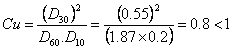< 50% passing #200                             coarse grained< 50% retained #4                                sand

< 5% passing #200 and  Cu<6 or  1>Cc>3  then soil SP

< 15% gravel then the soil is Poorly graded sand ………..ans.

3.25b   % passing #10 = 58                             LL=19

% passing #40              = 29                             PL=12

% passing #200            = 12                             PI = LL-PL=7<  35% passing #200                            Granular Material

From figure 3.18 left to right we obtain the soil is

A – 2 – 4 Silty or clayey gravel and sand (excellent to good) …..ans.

3.25b   % passing #10 = 62

% passing #40              = 24

% passing #200            = 4<  35% passing #200                            Granular Material

From figure 3.18 left to right we obtain the soil is

A – 1 – b Stone fragments, gravel, and sand (excellent to good) …..ans.